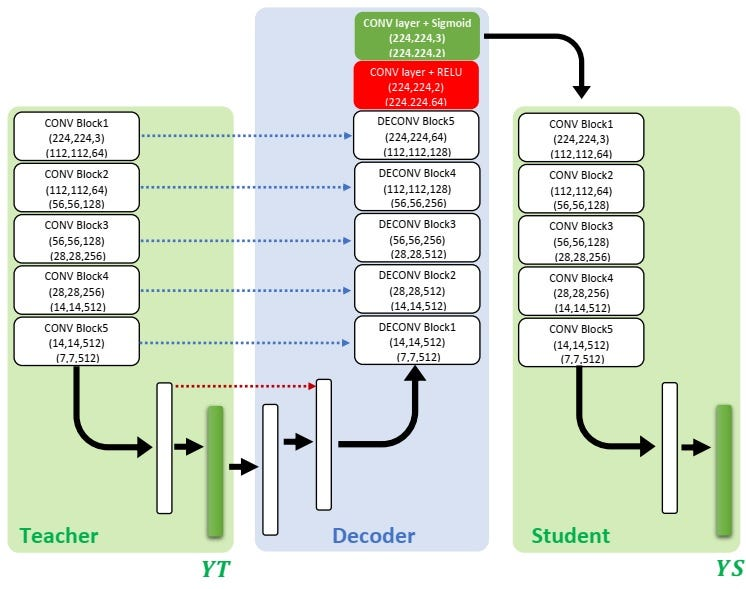# Teacher Student Architecture in Plant Disease Classification

Source: Deep Learning on Medium# Building the Teacher-Student Graph

In this next section, our focus will shift towards building the Teacher/Student graph architecture, as explained in the paper.

`# Teacher/Student graph.# Teacher ---> Decoder ---> Studentdef build_graph(input_shape = (224,224,3),nbr_of_classes=38,view_summary=False):#Teacher's graph. base_model1 = VGG16(include_top=False, weights='imagenet',input_shape = input_shape) x1_0 = base_model1.output x1_0 = Flatten(name='Flatten1')(x1_0) x1_1 = Dense(256, name='fc1',activation='relu')(x1_0)  x1_2 = classif_out_encoder1 = Dense(nbr_of_classes, name='out1', activation = 'softmax')(x1_1) #Decoder's graph. #Get Teacher's tensors for skip connection. pool5 = base_model1.get_layer('block5_pool').output conv5 = base_model1.get_layer('block5_conv3').output conv4 = base_model1.get_layer('block4_conv3').output conv3 = base_model1.get_layer('block3_conv3').output conv2 = base_model1.get_layer('block2_conv2').output conv1 = base_model1.get_layer('block1_conv2').output #Inverse fully connected Teacher's layers.  inv_x1_1 = Dense(256, name='inv_x1_1',activation='relu')(x1_2) merge_x1_1 = Add(name='merge_x1_1')([inv_x1_1,x1_1]) inv_x1_0 = Dense(7*7*512, name='x1_1',activation='relu')(merge_x1_1) reshaped_inv_x1_0 = Reshape((7, 7,512), name='')(inv_x1_0) inv_x1_0 = Add(name='merge_x1_0')([reshaped_inv_x1_0,pool5]) #DECONV Block1 up7 = Conv2D(512, 2, activation = 'relu', padding = 'same', kernel_initializer = 'he_normal')(UpSampling2D(size = (2,2))(inv_x1_0)) merge7 = concatenate([conv5,up7], axis = 3) conv7 = Conv2D(512, 3, activation = 'relu', padding = 'same', kernel_initializer = 'he_normal')(merge7) conv7 = Conv2D(512, 3, activation = 'relu', padding = 'same', kernel_initializer = 'he_normal')(conv7) #DECONV Block2 up8 = Conv2D(512, 2, activation = 'relu', padding = 'same', kernel_initializer = 'he_normal')(UpSampling2D(size = (2,2))(conv7)) merge8 = concatenate([conv4,up8], axis = 3) conv8 = Conv2D(512, 3, activation = 'relu', padding = 'same', kernel_initializer = 'he_normal')(merge8) conv8 = Conv2D(512, 3, activation = 'relu', padding = 'same', kernel_initializer = 'he_normal')(conv8) #DECONV Block13 up9 = Conv2D(256, 2, activation = 'relu', padding = 'same', kernel_initializer = 'he_normal')(UpSampling2D(size = (2,2))(conv8)) merge9 = concatenate([conv3,up9], axis = 3) conv9 = Conv2D(256, 3, activation = 'relu', padding = 'same', kernel_initializer = 'he_normal')(merge9) conv9 = Conv2D(256, 3, activation = 'relu', padding = 'same', kernel_initializer = 'he_normal')(conv9) #DECONV Block14 up10 = Conv2D(128, 2, activation = 'relu', padding = 'same', kernel_initializer = 'he_normal')(UpSampling2D(size = (2,2))(conv9)) merge10 = concatenate([conv2,up10], axis = 3) conv10 = Conv2D(128, 3, activation = 'relu', padding = 'same', kernel_initializer = 'he_normal')(merge10) conv10 = Conv2D(128, 3, activation = 'relu', padding = 'same', kernel_initializer = 'he_normal')(conv10) #DECONVBlock15 up11 = Conv2D(64, 2, activation = 'relu', padding = 'same', kernel_initializer = 'he_normal')(UpSampling2D(size = (2,2))(conv10)) merge11 = concatenate([conv1,up11], axis = 3) conv11 = Conv2D(64, 3, activation = 'relu', padding = 'same', kernel_initializer = 'he_normal')(merge11) conv11 = Conv2D(64, 3, activation = 'relu', padding = 'same', kernel_initializer = 'he_normal')(conv11) #Reconstructed image refinement conv11 = Conv2D(2, 3, activation = 'relu', padding = 'same', kernel_initializer = 'he_normal')(conv11) mask = conv11 = Conv2D(3, 1, activation = 'sigmoid',name='Mask')(conv11)#Graphe of Student base_model2 = VGG16(include_top=False, weights='imagenet',input_shape = (224,224,3)) x2_0 = base_model2(mask) x2_0 = Flatten(name='Flatten2')(x2_0) x2_1 = Dense(256, name='fc2',activation='relu')(x2_0)  classif_out_encoder2 = Dense(nbr_of_classes, name='out2',activation='softmax')(x2_1)#Get Teacher/Student Model model = Model(input = base_model1.input, output = [classif_out_encoder1,classif_out_encoder2])if(view_summary): print(mode.summary())#Compile the mode to use multi-task learning losses = { "out1": 'categorical_crossentropy', "out2": 'categorical_crossentropy' } alpha=0.4 lossWeights = {"out1": alpha, "out2": (1.0-alpha)} model.compile(optimizer=optimizers.SGD(lr=1e-4, momentum=0.9), loss=losses, loss_weights=lossWeights,metrics = ['accuracy'])return model`

After building the graph method for our Teacher/Student architecture, we’ll need several helper functions.

Preprocess the images w/ the correct size

`def preprocess_image(image_path, image_size = (224, 224)): img = image.load_img(image_path, target_size=image_size) img = image.img_to_array(img) img = np.expand_dims(img, axis=0) img = preprocess_input(img) return img`

Build the visualization graph w/ the loaded weights

`def build_visualization(model_weights_path): model = build_graph() model.load_weights(model_weights_path) layer_name ='Mask' NewInput = model.get_layer(layer_name).output visualization = K.function([model.input], [NewInput]) return visualization`

Reduce the channels and create the heatmap

`def reduce_channels_sequare(heatmap): channel1 = heatmap[:,:,0] channel2 = heatmap[:,:,1] channel3 = heatmap[:,:,2] new_heatmap = np.sqrt((channel1*channel1)+(channel2*channel2)+(channel3*channel3)) return new_heatmap`

Postprocess the visualizations w/ a threshold value and return the new heatmap

`def postprocess_vis(heatmap1, threshold = 0.9): heatmap = heatmap1.copy() heatmap = (heatmap - heatmap.min())/(heatmap.max() - heatmap.min()) heatmap = reduce_channels_sequare(heatmap) heatmap = (heatmap - heatmap.min())/(heatmap.max() - heatmap.min()) heatmap[heatmap <= threshold] = 0 heatmap = heatmap*255 return heatmap`

Set up the visualization schema w/ the image path and output folder

`def visualize_image(visualization, image_path, out_folder): base = os.path.basename(image_path) image_name = os.path.splitext(base) img = preprocess_image(image_path) vis = visualization([img])*255 heatmap = postprocess_vis(vis) vis_path = os.path.join(out_folder, image_name+'_vis.jpg') cv2.imwrite(vis_path, vis) heatmap_path = os.path.join(out_folder, image_name+'_heatmap.jpg') cv2.imwrite(heatmap_path, heatmap)`

Visualize the images given the image folder path and output folder

`def visualize_folder(visualization, images_folder, out_folder): if not os.path.exists(out_folder): os.makedirs(out_folder for path in os.listdir(images_folder): print(path) image_path = os.path.join(images_folder, path) visualize_image(visualization, image_path, out_folder)`

Finally, we’ll build out the visualization and return the results

`visualization = build_visualization(model_weights_path)visualize_folder(visualization, images_folder, out_folder)`